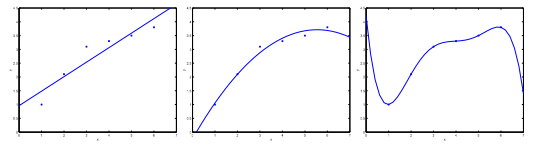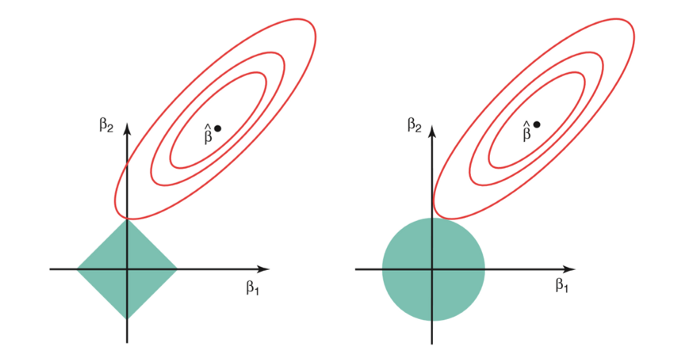# Elastic Net Regression

What is regression?

Regression is a set of statistical processes for estimating the relationships between a dependent or target variable and one or more independent or feature variables. The most common form of regression used is linear regression, in which we find a line or its n-dimensional counterpart that most closely fits the data according to a specific mathematical criterion.

Regularization

Real data is noisy and overfitting on it results in bad future predictions. So, to prevent such a problem we use regularization while training our regression models.

To understand why regularization is important take a look at the below picture.Here, we see three prediction curves for the same dataset. The first curve is underfitted to our dataset, the third curve is overfitted and the middle curve is arguably the best fit.

Underfitting can be prevented by making a more complicated model, for example by taking polynomial features.

But to prevent overfitting we either need more data, which is an expensive process and thus not preferred, or we can use regularization which penalizes the model when it has high coefficient values and therefore reduces the complexity of our model.

There are various ways to introduce regularization in our model. The most common ones are L1 regularization and L2 regularization.

L1 and L2 Regularization

The basic essence of regularization is to add a penalty term to our loss function and this penalty term should increase as the values of coefficients are increased.

L1 and L2 regularization methods are based on this basic concept only.

In L1 regularization we add the sum of the absolute values of coefficients.

Similarly, in L2 regularization we add the sum of the squares of the values of the coefficients to our loss function.

We can understand the effects of these with the help of the below visualization.The first graph is of the L1 regularization and the second graph is of L2 regularization.

The contour plots represent our loss function. The regularization term restricts our model to minimize the loss function by keeping the values of the coefficients in a range (represented in the green/blue figure).

L2 regularization minimizes the size of all coefficients, although it prevents any coefficients from being removed from the model. On the other hand, L1 regularization minimizes the size of all coefficients and allows some coefficients to be minimized to the value zero, which removes the predictor from the model.

Elastic Net Regression

There are pros and cons of both L1 and L2 regularization. So, when both L1 and L2 regularization fail to improve our regression we include both of their penalties in a single model which is called Elastic Net Regularization.

Elastic Net Regression is a penalized regression model that includes both L1 and L2 penalties in the loss function while training.

A new hyperparameter is thus introduced which manages the ratio of each of the L1 or L2 penalty to be included.

Mathematically, we can write

Elastic    Net Penalty = (alpha*L1 Penalty) + ((1-alpha)*L2 Penalty)

where,

L1 Penalty = sum of absolute value of coefficients

L2 Penalty = sum of squares of the values of the coefficients

alpha is our elastic net hyperparameter

We can include the above penalty to our Regression model's cost function.

Elastic Net Loss = Regular Loss + (lambda*Elastic Net Penalty)

where,

Regular Loss is our mean squared error

lambda is our regularization hyperparameter

The benefit of Elastic Net Regression is that it allows a balance of both L1 and L2 penalties which can result in better performance than a model with only either one of those.#### More Articles of Aniket Sharma:

Name Views Likes
Pyperclip: Installation and Working 991 2
Number Guessing Game using Python 683 2
Pyperclip: Not Implemented Error 1033 2
Hangman Game using Python 16821 2
Using Databases with CherryPy application 1676 2
nose: Working 509 2
pytest: Working 512 2
Open Source and Hacktoberfest 868 2
Managing Logs of CherryPy applications 1005 2
Top 20 Data Science Tools 684 2
Ajax application using CherryPy 799 2
REST application using CherryPy 664 2
On Screen Keyboard using Python 5532 2
Elastic Net Regression 816 2
US Presidential Election 2020 Prediction using Python 795 2
Sound Source Separation 1165 2
URLs with Parameters in CherryPy 1635 2
Testing CherryPy application 637 2
Handling HTML Forms with CherryPy 1449 2
Applications of Natural Language Processing in Businesses 511 2
NetworkX: Multigraphs 649 2
Tracking User Activity with CherryPy 1404 2
Introduction to NetworkX 633 2
TorchServe - Serving PyTorch Models 1306 2
Fake News Detection Model using Python 735 2
Keeping Home Routers secure while working remotely 484 2
Email Slicer using Python 2998 2
NetworkX: Creating a Graph 1111 2
Best Mathematics Courses for Machine Learning 551 2
Hello World in CherryPy 681 2
Building dependencies as Meson subprojects 979 2
Vehicle Detection System 1081 2
NetworkX: Examining and Removing Graph Elements 608 2
Handling URLs with CherryPy 537 2
PEP 8 - Guide to Beautiful Python Code 759 2
NetworkX: Drawing Graphs 624 2
Mad Libs Game using Python 645 2
Hosting Cherry applications 613 2
Top 5 Free Online IDEs of 2020 867 2
pytest: Introduction 535 2
Preventing Pwned and Reused Passwords 582 2
Contact Book using Python 2095 2
Introduction to CherryPy 547 2
nose: Introduction 505 2
Text-based Adventure Game using Python 3002 2